# Gimme A Break

## Adding/Subtracting and an Intro to Multiplying/Dividing Fractions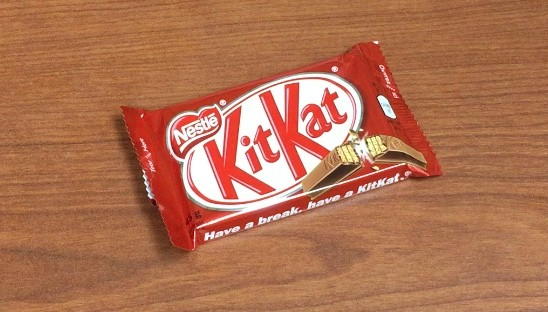A colleague and good friend, Dave Burke, reached out to me recently for some ideas around adding and subtracting fractions. In particular, he was interested in trying to make the topic a little more enjoyable by adding some context. This was a challenge I was really eager to work on because working with fractions is such a sore spot for so many students and their teachers. In the past, I tried to help make dividing fractions less abstract by using the idea of dividing chocolate bars. With this in mind, I figured using chocolate bars for adding and subtracting would be a good starting point to move towards multiplication and division later.

Here are some expectations from the Grade 7 Ontario math curriculum that we’ll be aiming to touch on:

• Grade 7, NS2.01 – divide whole numbers by simple fractions and by decimal numbers to hundredths, using concrete materials (e.g., divide 3 by ½ using fraction strips; divide 4 by 0.8 using base ten materials and estimation);
• Grade 7, NS2.02 – use a variety of mental strategies to solve problems involving the addition and subtraction of fractions and decimals (e.g., use the commutative property: 3 x 2/5 x 1/3 = 3 x 1/3 x 2/5, which gives 1 x 2/5 = 2/5 ; use the distributive property: 16.8 ÷ 0.2 can be thought of as (16 + 0.8) ÷ 0.2 = 16 ÷ 0.2 + 0.8 ÷ 0.2, which gives 80 + 4 = 84);
• Grade 7, NS2.07 – add and subtract fractions with simple like and unlike denominators, using a variety of tools (e.g., fraction circles, Cuisenaire rods, drawings, calculators) and algorithms;
• Grade 7, NS2.08 – demonstrate, using concrete materials, the relationship between the repeated addition of fractions and the multiplication of that fraction by a whole number (e.g., 1/2 + 1/2 + 1/2 = 3 x 1/2);

I was surprised when I reviewed the grade 7 curriculum to find that the idea of dividing whole numbers by simple fractions was listed before adding and subtracting fractions. The more I thought about this, the more I like it. However, I stuck to adding/subtracting first.

So, let’s get started…

Show the students the video below:

After watching the video, I have students take 30 seconds to discuss with their elbow partners what question(s) come to mind before sharing out with the group. I usually give them an open response question in Knowledgehook Gameshow to ensure all voices can be heard:

When the list of questions is exhausted, I then show them act 2…

## Task 1, Act 2 – Revealing Some Information

Show the students the video below:

Students quickly come to the conclusion that the question I am asking here is…

How many pieces of a whole KitKat bar are there?

I’ve been using Knowledgehook Gameshow as a way to keep my lessons interactive almost daily. The best feature is the upload solution tool (not available when embedded in websites) that allows me to share out student work instantly over the projector. I find using Gameshow makes it even easier for me to be the facilitator of the learning rather than the gatekeeper of knowledge. Typically, the solutions students share is enough to consolidate most tasks and I can minimize the amount of direct instruction I must deliver.

## Task 1, Act 3 – The Solution

It is always nice to be able to show students the solution for a little celebration. This is where I see Gameshow could make an improvement. Have the option to add a “solution page” where you can insert media, rather than me having to open a video file or run a slide deck.

Then, we continue on, slowly raising the bar on the complexity of the problems, but keeping the context the same.

## Task 2, Act 2 – Revealing New Information

Then, show students this video:

Students can then evaluate the expression.

## Task 3, Act 1 – Introducing the Problem

Ask students to talk to their neighbours about what question we might ask here. The goal is to have them realize that they are going to need to do some addition and subtraction of fractions.

## Task 3, Act 2 – Revealing Some Information

Students can then evaluate the expression in Knowledgehook Gameshow.

Here are a few Knowledgehook Gameshow questions that might be useful for some practice prior to moving on:

# Task 4 – Multiplying Whole Numbers By Simple Fractions

## Task 4, Act 1 – Introducing the Problem

Ask students to talk to their neighbours about what question we might ask here. Some students might say we are adding, which is great. However, I wonder if any students will notice that we are not only adding, but we are doing repeated addition (multiplication).

Students can then give their take on what they think will happen.

In order to “show” students this, play this clip:

## Task 4, Act 2 – Introducing the Problem

Now, it’s time for students to take this new idea and run with it. Show them this image: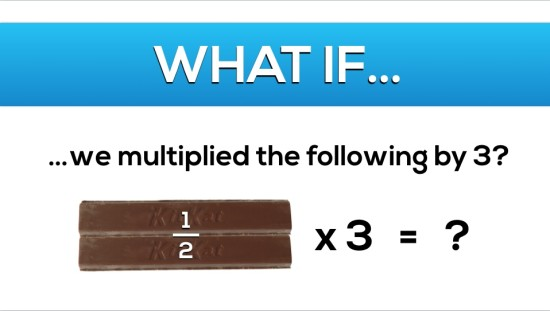Let students chat it out and then, when they are ready, they can give an open response of their thinking.

After exploring some of the open responses submitted by students, we can ask them to determine the answer to the problem.

Show your students the video below:

# Task 5 – Multiplying Whole Numbers By Simple Fractions

## Task 5, Act 1 & 2 – Introducing Problem And Giving Information

Now, let’s give students an opportunity to try another problem involving multiplication. Show them this image: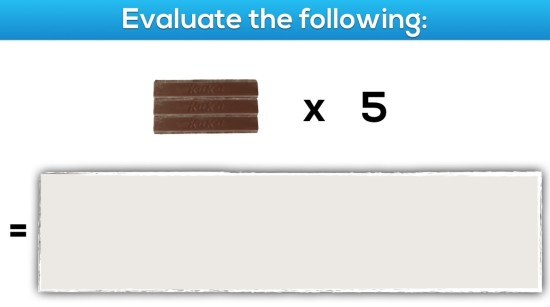Give them an opportunity to solve the problem by evaluating and interact with their classmates.

Show students the solution video:

# Task 6 – Dividing Whole Numbers By Simple Fractions

## Task 6, Act 1 – Introducing Problem

Show students this video:

Students are then given some time to discuss what they think might be going on here and then they share out their thinking.

Now, you can show the students some more information to get their wheels turning:

Then, students can solve and share out their solutions.

Then, you can show students the video of the answer:

# Task 7 – Dividing Whole Numbers By Simple Fractions

## Task 7, Act 1 & 2 – Introducing Problem and Revealing Information

Show students this image: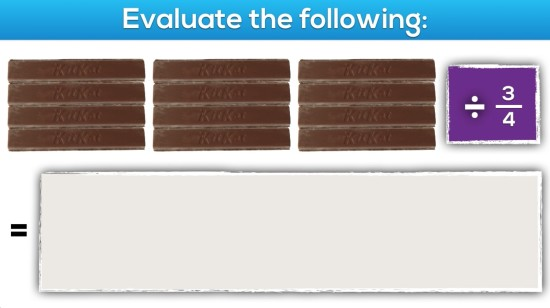Show students this video:That’s it for now, but I do have a huge bag full of other chocolate bars that I intend to eventually use to make more problems involving fractions. Let me know how you like this one so I know if it is worth putting in the extra effort.

If you’re interested, you can try the full Knowledgehook Gameshow here or, you can copy the entire gameshow to your own Free Gameshow Account via the share link below:

## New to Using 3 Act Math Tasks?

Download the 2-page printable 3 Act Math Tip Sheet to ensure that you have the best start to your journey using 3 Act math Tasks to spark curiosity and fuel sense making in your math classroom!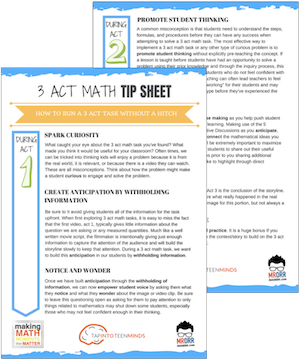## Share With Your Learning Community:I’m Kyle Pearce and I am a former high school math teacher. I’m now the K-12 Mathematics Consultant with the Greater Essex County District School Board, where I uncover creative ways to spark curiosity and fuel sense making in mathematics. Read more.

Access Other Real World Math Tasks

## Search More 3 Act Math Tasks

Spark Curiosity!